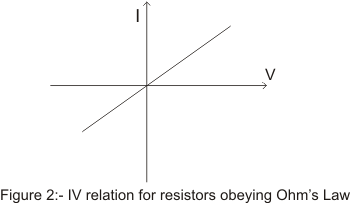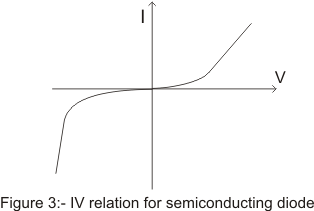# Ohm's Law & resistance<

## Ohm's Law and Resistance

• Ohm's law is the relation between the potential difference applied to the ends of the conductor and current flowing through the conductor.This law was expressed by George Simon Ohm in 1826
• Statement of Ohm's Law
'if the physical state of the conductor (Temperature and mechanical strain etc.) remains unchanged ,then current flowing through a conductor is always directly proportional to the potential difference across the two ends of the conductor
Mathematically
V α I
or
V=IR                    (6)
Where constant of proportionality R is called the electric resistance or simply resistance of the conductor
• Value of resistance depends upon the nature ,dimension and physically dimensions of the conductor
• Ohm's Law can be deducted using drift velocity relation as given in equation -3 .Thus from the equation
vd=(eE/m)τ
but Now E=V/l
Therefore
vd=(eV/ml)τ
Also I=neAvd
Substituting the value of vd in I relation
I=(ne2Aτ/ml) V                    (7)
or V/I=(ml/ne2Aτ)=R a constant for a given conductor
Thus
V=IR
Mathematical expression of Ohm's Law
From Ohm's Law
V=IR or R=V/I                    (8)
Thus electric resistance is the ratio of potential difference across the two ends of conductor and amount of current flowing through the conductor
• electric resistance of a conductor is the obstruction offered by the conductor to the flow of the current through it.
• SI unit of resistance is ohm (Ω) where
1 Ohm=1 volt/1 Ampere
or 1Ω=1VA-1
• Dimension of resistance is [ML2T-3A-2]

## Current Voltage relations & Limitations

• We know that current through any electrical device such as resistors depends on potential difference between the terminals
• Devices obeying ohm's law follow a linear relationship between current following and potential applied where current is directly proportional to voltage applied .Graphical relation between V and I is shown below in figure• Graph for a resistor obeying ohm's law is a straight line through the origin having some finite slope
• There are many electrical devices that does not obey the ohm's law and current may depends on voltage in more complicated ways.Such devices are called non-ohmic devices for examples vacuum tubes,semiconductor diodes ,transistors etc
• Consider the case of a semi conductor junction diode which are used to convert alternating current to direct current and are used to perform variety of logic functions is a non=ohmic device
• Graphical voltage relation for a diode is shown below in the figure• Figure clearly shows a non linear dependency of current on voltage and diode clearly does not follow the ohm's w
• When a device does not follow obey ohm's law,it has non linear voltage -current relation and the quantity V/I is no longer a constant however ratio is still known as resistance which now varies with current
• In such cases we define a quantity dV/dI known as dynamic resistance which expresses the relation between small change in current and resulting change in voltage
• Thus for non-ohmic electrical devices resistance is not constant for different values of V and I

Latest Articles
Synthetic Fibres and Plastics Class 8 Practice questions

Class 8 science chapter 5 extra questions and Answers

Mass Calculator

3 Fraction calculator

Garbage in Garbage out Extra Questions7